﻿ 基于非线性有限元方法的单点系泊系统的优化设计研究 The Design of Single Point Mooring System with Nonlinear Finite Element Method

Advances in Applied Mathematics
Vol.06 No.04(2017), Article ID:21454,19 pages
10.12677/AAM.2017.64066

The Design of Single Point Mooring System with Nonlinear Finite Element Method

Shuai Miao, Jianyu Wu, Rongrong Zhang, Zhuo Zhang

School of Mathematical Sciences, Inner Mongolia University, Hohhot Inner Mongolia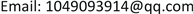Received: Jul. 1st, 2017; accepted: Jul. 17th, 2017; published: Jul. 21st, 2017ABSTRACT

Based on the principle of statics, this paper analyzes the force analysis of six large parts in mooring system. A series of equations of physics are listed. Finally, we derive the nonlinear differential equation model about mooring system, which is solved by iterative method. According to the structure of anchor chain, the corresponding structure method of finite element method is adopted. By using the nonlinear finite element method, this paper obtains the anchor chain equation of mooring system. To calculate the mooring system without lifting angle limit boundary condition, we analyze the stress of anchor, which cannot be dragged easily, and we try to keep the condition of vertical steel drum. We find the influence of wind power, water power, steel pipe, angle, shape, anchor buoy and draught of the swimming area, to ensure the stable condition of the system.

Keywords:Static Analysis Principle, Finite Element, Mooring System, Differential Equations, Nonlinear ProgrammingCopyright © 2017 by authors and Hans Publishers Inc.1. 研究背景及目的

40多年来，随着近海石油勘探开发和海上运输业的发展，单点系泊技术的发展十分迅速。目前，这种技术已作为一种成熟的海上中转、仓储、过驳技术被世界各国竞相采用。首先，单点作为海上中转终端，通过这种“浮动的码头”，就可以供大型船舶系泊和装卸原油或矿砂，充分发挥了大型船舶运输的优越性。其次，单点作为海上油田采油终端，起到了“浮动油库”的作用，是深海、遥远油田上经济而且先进的储输手段。可以这样说，单点系泊技术为海洋石油开采和海上运输中转的历史翻开了崭新的一页，极大地推动了海洋石油开采业和石油化工业的发展。

1) 建立微分方程组模型，用数值计算的方法分析不同风速下，钢桶和各节钢管的倾斜角度，锚链形状，浮标的吃水深度和游动区域，

2) 第二问在问题一的假设下，调节重物球的质量，使得钢桶的倾斜角不超过5度，锚链与海床的夹角不超过16度。

3)增加了海水的速度，考虑风力、水速和水深等因素的影响并且分析在不同的风速下钢桶，钢管的倾斜角度，锚链形状，浮标的吃水深度和游动区域。

2. 模型的假设

1) 假设本问题所使用的数据真实有效，具有统计分析价值。

2) 前期不考虑海啸、台风等特殊天气对于系泊系统的影响。

3) 假设系泊系统中所有部件都处于理想的平衡状态。

4) 假设系泊系统中各部件连接处光滑。

5) 假定浅海底为水平面，且锚链不发生弹性形变；

6) 假设系泊系统中每个部件所有点的运动情况都相同，大小和形状对研究问题的影响很小，可视为质点。

3. 符号说明

4. 模型的建立及求解

4.1. 问题一

4.1.1. 受力分析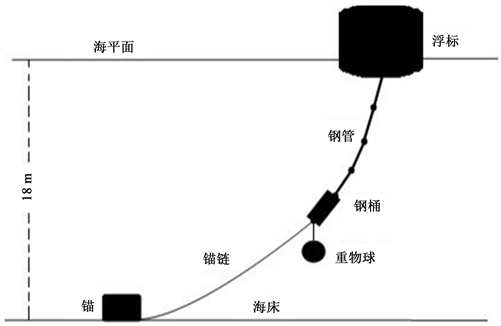Figure 1. Schematic diagram of mooring system transmission node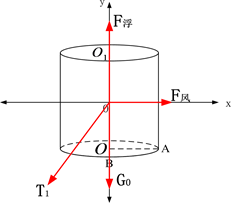Figure 2. Force analysis chart of buoy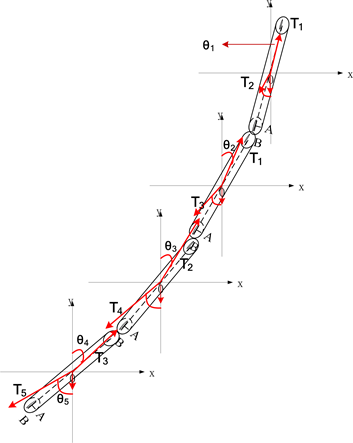Figure 3. Four sections of steel pipe analysis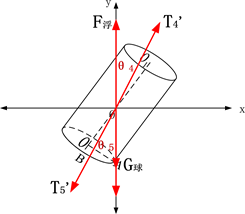Figure 4. Steel drum and equipment force analysis diagram

4.1.2. 模型建立：静力学模型的建立 

4.1.3. 求解模型

${h}^{\prime }$ 表示浮标的吃水深度，h表示浮标总高度，Fx表示风给浮标的水平力，则有：

${F}_{x}=0.625{v}^{\text{2}}S\left(\theta ,h\right)=0.625×2r\left(h-{h}^{\prime }\right){v}^{\text{2}}$ (1-1)

${F}_{f}=\rho g\text{π}{r}^{2}{h}^{\prime }$ (1-2)

${F}_{x}={T}_{1}\mathrm{sin}{\theta }_{1},\text{\hspace{0.17em}}{F}_{f}={T}_{1}\mathrm{cos}{\theta }_{1}+{G}_{0}$ (1-3)

${T}_{1}\mathrm{sin}{\theta }_{1}={T}_{2}\mathrm{sin}{\theta }_{2},\text{\hspace{0.17em}}{T}_{1}\mathrm{cos}{\theta }_{1}={T}_{2}\mathrm{cos}{\theta }_{2}+{G}_{1}$ (1-4)

${T}_{2}\mathrm{sin}{\theta }_{2}={T}_{3}\mathrm{sin}{\theta }_{3},\text{\hspace{0.17em}}{T}_{2}\mathrm{cos}{\theta }_{2}={T}_{3}\mathrm{cos}{\theta }_{3}+{G}_{2}$ (1-5)

${T}_{3}\mathrm{sin}{\theta }_{3}={T}_{4}\mathrm{sin}{\theta }_{4},\text{\hspace{0.17em}}{T}_{3}\mathrm{cos}{\theta }_{3}={T}_{4}\mathrm{cos}{\theta }_{4}+{G}_{3}$ (1-6)

${T}_{4}\mathrm{sin}{\theta }_{4}={T}_{5}\mathrm{sin}{\theta }_{5},\text{\hspace{0.17em}}{T}_{4}\mathrm{cos}{\theta }_{4}={T}_{5}\mathrm{cos}{\theta }_{5}+{G}_{4}$ (1-7)

${T}_{5}\mathrm{sin}{\theta }_{5}={T}_{6}\mathrm{sin}{\theta }_{6},\text{\hspace{0.17em}}{T}_{5}\mathrm{cos}{\theta }_{5}={T}_{6}\mathrm{cos}{\theta }_{6}+{G}_{T}+{G}_{q}$ (1-8)

${T}_{6}$ 表示锚链与钢筒之间的相互作用力， ${\theta }_{6}$ 表示钢桶与垂直方向的夹角。GT为钢筒自重，Gq为重物球重力。

$22.05×\mathrm{cos}{\theta }_{6}+1×\mathrm{cos}{\theta }_{5}+1×\mathrm{cos}{\theta }_{4}+1×\mathrm{cos}{\theta }_{3}+1×\mathrm{cos}{\theta }_{2}+1×\mathrm{cos}{\theta }_{1}+{h}^{\prime }=18$ (1-9)

$W={\left(22.05×\mathrm{cos}{\theta }_{6}+1×\mathrm{cos}{\theta }_{5}+1×\mathrm{cos}{\theta }_{4}+1×\mathrm{cos}{\theta }_{3}+1×\mathrm{cos}{\theta }_{2}+1×\mathrm{cos}{\theta }_{1}+{h}^{\prime }-18\right)}^{2}$ (1-10)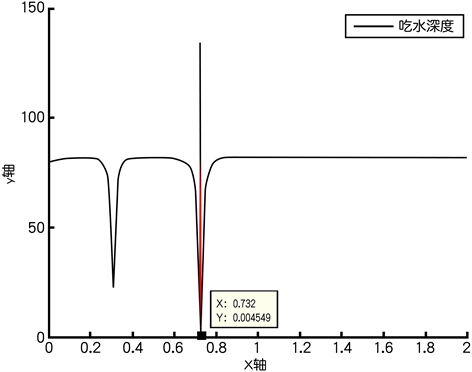Figure 5. The depth of the draft at 12 m/s (the static water)

$z=\frac{{T}_{6}}{\omega }\left(ch\frac{x}{a}-1\right)$ (1-11)

$z=\frac{{T}_{6}}{70}\left(ch\frac{70x}{{T}_{6}}-1\right);\text{\hspace{0.17em}}s=\frac{{T}_{6}}{70}sh\frac{70x}{{T}_{6}}$ (1-12)

$s=\frac{{T}_{6}}{70}sh\frac{70x}{{T}_{6}}=22.05⇒{x}_{0}=\frac{{T}_{6}}{70}\text{asinh}\left(\frac{70×22.05}{{T}_{6}}\right)$ (1-13)

${z}_{0}=\frac{{T}_{6}}{\omega }\left(ch\frac{{x}_{0}}{a}-1\right)$ (1-14)

4.1.4. 系泊系统的锚链的非线性有限元计算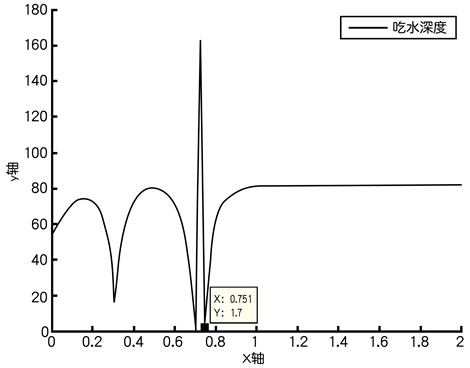Figure 6. The depth of the draft at 24 m/s (the static water)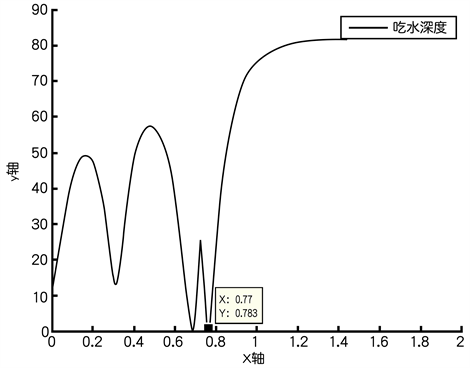Figure 7. The depth of the draft at 36 m/s (the static water)

$\tau =\frac{\text{d}x}{\text{d}s}i+\frac{\text{d}y}{\text{d}s}j+\frac{\text{d}z}{\text{d}s}k={\alpha }_{1}i+{\alpha }_{2}j+{\alpha }_{3}k$ (1-15)

$n=\rho \left(\frac{{\text{d}}^{2}x}{\text{d}{s}^{2}}i+\frac{{\text{d}}^{2}y}{\text{d}{s}^{2}}j+\frac{{\text{d}}^{2}z}{\text{d}{s}^{2}}k\right)={\beta }_{1}i+{\beta }_{2}j+{\beta }_{3}k$ (1-16)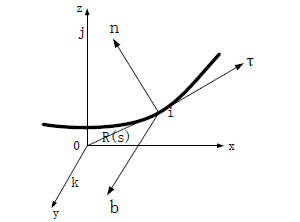Figure 8. Two kinds of coordinate system

$b=\tau \cdot n={\gamma }_{1}i+{\gamma }_{2}j+{\gamma }_{3}k$

$\rho ={\left[\left(\frac{{\text{d}}^{2}x}{\text{d}{s}^{2}}\right)i+\left(\frac{{\text{d}}^{2}y}{\text{d}{s}^{2}}\right)j+\left(\frac{{\text{d}}^{2}z}{\text{d}{s}^{2}}\right)k\right]}^{1/2}$ (1-17)

$\tau \cdot \tau =\sum _{i=1}^{3}\text{ }\text{ }{\alpha }_{i}=1$$n\cdot n=\sum _{i=1}^{3}{\beta }_{i}=1$$b\cdot b=\sum _{i=1}^{3}{\gamma }_{i}=1$ (1-18)

$\tau \cdot n=\sum _{i=1}^{3}{\alpha }_{i}{\beta }_{i}=0$$n\cdot b=\sum _{i=1}^{3}{\beta }_{i}{\gamma }_{i}=0$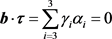(1-19)

$\left[\begin{array}{c}\tau \\ n\\ b\end{array}\right]=\left[\begin{array}{ccc}{\alpha }_{1}& {\alpha }_{2}& {\alpha }_{3}\\ {\beta }_{1}& {\beta }_{2}& {\beta }_{3}\\ {\gamma }_{1}& {\gamma }_{2}& {\gamma }_{3}\end{array}\right]\left[\begin{array}{c}i\\ j\\ k\end{array}\right]$ (1-20)

$\left[\begin{array}{c}\tau \\ n\\ b\end{array}\right]=\left[\begin{array}{ccc}{\alpha }_{1}& {\beta }_{1}& {\gamma }_{1}\\ {\alpha }_{2}& {\beta }_{2}& {\gamma }_{2}\\ {\alpha }_{3}& {\beta }_{3}& {\gamma }_{3}\end{array}\right]\left[\begin{array}{c}i\\ j\\ k\end{array}\right]$ (1-21)

$X=ui+vj+wk$ (1-22)

$\tau nb$ 能这么表示：

$X=\xi \tau +\eta n+\zeta b$ (1-23)

$\left[\begin{array}{c}\xi \\ \eta \\ \zeta \end{array}\right]=\left[\begin{array}{ccc}{\alpha }_{1}& {\alpha }_{2}& {\alpha }_{3}\\ {\beta }_{1}& {\beta }_{2}& {\beta }_{3}\\ {\gamma }_{1}& {\gamma }_{2}& {\gamma }_{3}\end{array}\right]\left[\begin{array}{c}u\\ v\\ w\end{array}\right]$ (1-24)

$\left[B\right]=\left[\begin{array}{ccc}{\alpha }_{1}& {\alpha }_{2}& {\alpha }_{3}\\ {\beta }_{1}& {\beta }_{2}& {\beta }_{3}\\ {\gamma }_{1}& {\gamma }_{2}& {\gamma }_{3}\end{array}\right]$ ，其有如下性质：

${\left[B\right]}^{\text{T}}\left[B\right]=\left[I\right]$$\left[B\right]={\left[B\right]}^{-1}$ (1-25)

$\left[I\right]$ 表示单位矩阵。

${f}_{1}=\left(T+\frac{1}{2}\frac{\text{d}T}{\text{d}s}\text{d}s\right)\left(t+\frac{1}{2}\frac{\text{d}t}{\text{d}s}\text{d}s\right)$ (1-26)

${f}_{2}=-\left(T-\frac{1}{2}\frac{\text{d}T}{\text{d}s}\text{d}s\right)\left(t-\frac{1}{2}\frac{\text{d}t}{\text{d}s}\text{d}s\right)$ (1-27)

$v={v}_{w}-{v}_{c}$ (1-28)

$a={a}_{w}-{a}_{c}$ (1-29)

$T$$T$ ，锚链的两个顶端分别产生的拉力时 ${T}_{1},{T}_{2}$ ，即：

$\begin{array}{l}{T}_{1}=\left(T+\frac{1}{2}\frac{\text{d}T}{\text{d}s}\text{d}s\right)\left(\tau +\frac{1}{2}\frac{\text{d}\tau }{\text{d}s}\text{d}s\right)\\ {T}_{2}=-\left(T-\frac{1}{2}\frac{\text{d}T}{\text{d}s}\text{d}s\right)\left(\tau -\frac{1}{2}\frac{\text{d}\tau }{\text{d}s}\text{d}s\right)\end{array}$ (1-30)

$\begin{array}{c}\text{d}T={T}_{1}-{T}_{2}=\left(T+\frac{1}{2}\frac{\text{d}T}{\text{d}s}\text{d}s\right)\left(\tau +\frac{1}{2}\frac{\text{d}\tau }{\text{d}s}\text{d}s\right)-\left(T-\frac{1}{2}\frac{\text{d}T}{\text{d}s}\text{d}s\right)\left(\tau -\frac{1}{2}\frac{\text{d}\tau }{\text{d}s}\text{d}s\right)\\ =\left(\frac{\text{d}T}{\text{d}s}\tau +T\frac{\text{d}\tau }{\text{d}s}\right)\text{d}s=\left(\frac{\text{d}T}{\text{d}s}\tau +\frac{T}{\rho }n\right)\text{d}s\end{array}$ (1-31)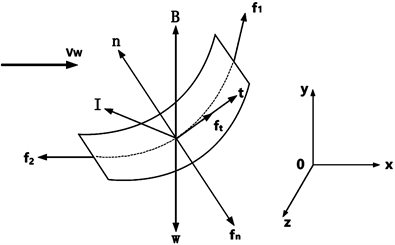Figure 9. Anchor chain stress analysis chart

${f}_{N}+{f}_{\tau }+\left(W-B\right)+\text{d}T+I=0$ (1-32)

${f}_{N}\text{d}s=\frac{1}{2}{\rho }_{w}{C}_{Dn}D|{v}_{N}|{v}_{N}\text{d}s$ (1-33)

${f}_{\tau }\text{d}s=\frac{1}{2}{\rho }_{w}{C}_{Dr}\text{π}D|{v}_{\tau }|{v}_{\tau }\text{d}s$ (1-34)

$W-B=\left({\rho }_{c}-{\rho }_{w}\right)A\text{d}sg$ (1-35)

$I=-{\rho }_{C}A\text{d}s{a}_{\xi }+{C}_{m}{\rho }_{w}A\text{d}s\left({a}_{w}-{a}_{c}\right)$ (1-36)

${\rho }_{c}A\text{d}s{a}_{c}-{C}_{m}{\rho }_{w}A\text{d}s\left({a}_{w}-{a}_{c}\right)$ $=\left({\rho }_{c}-{\rho }_{w}\right)A\text{d}sg+\frac{1}{2}{\rho }_{w}{C}_{D}{}_{\tau }\text{π}D|{v}_{r}|{v}_{r}\text{d}s+\frac{1}{2}{\rho }_{w}{C}_{Dn}D|{v}_{N}|{v}_{N}\text{d}s+\text{d}T$ (1-37)

${\rho }_{c}A{a}_{c}-{C}_{m}{\rho }_{w}A\left({a}_{w}-{a}_{c}\right)=\left({\rho }_{c}-{\rho }_{w}\right)Ag+\frac{1}{2}{\rho }_{w}{C}_{D\tau }\text{π}D|{v}_{r}|{v}_{r}+\frac{1}{2}{\rho }_{w}{C}_{Dn}D|{v}_{N}|{v}_{N}+\frac{\text{d}T}{\text{d}s}$ (1-38)

$\left(1+{C}_{m}\right){\rho }_{c}A{a}_{c}={C}_{m}{\rho }_{w}A{a}_{w}+\left({\rho }_{c}-{\rho }_{w}\right)Ag+\frac{1}{2}{\rho }_{w}{C}_{D\tau }\text{π}D|{v}_{r}|{v}_{r}+\frac{1}{2}{\rho }_{w}{C}_{Dn}D|{v}_{N}|v+\frac{\text{d}T}{\text{d}s}$ (1-39)

${f}_{N}+{f}_{r}+\left(W-B\right)+\text{d}T=0$ (1-40)

$\left({\rho }_{c}-{\rho }_{w}\right)Ag+\frac{1}{2}{\rho }_{w}{C}_{D\tau }\text{π}D|{v}_{r}|{v}_{\tau }+\frac{1}{2}{\rho }_{w}{C}_{Dn}D|{v}_{N}|{v}_{N}+\frac{\text{d}T}{\text{d}s}=0$ (1-41)

$\left\{\begin{array}{l}\left({\rho }_{c}-{\rho }_{w}\right)Ag\cdot t+\frac{1}{2}{\rho }_{w}{C}_{D\tau }\text{π}D|{v}_{r}|{v}_{\tau }+\frac{\text{d}T}{\text{d}s}=0\\ \left({\rho }_{c}-{\rho }_{w}\right)Ag\cdot n+{f}_{n}\cdot n+\frac{T}{\rho }=0\\ \left({\rho }_{c}-{\rho }_{w}\right)Ag\cdot b+{f}_{n}\cdot b=0\end{array}$ (1-42)

$\left\{\begin{array}{l}\left({\rho }_{c}-{\rho }_{w}\right)Ag\cdot t+\frac{1}{2}{\rho }_{w}{C}_{D\tau }\text{π}D|{v}_{r}|{v}_{\tau }+\frac{\text{d}T}{\text{d}s}=0\\ \left({\rho }_{c}-{\rho }_{w}\right)Ag\cdot n+\frac{1}{2}{\rho }_{w}{C}_{Dn}D|{v}_{n}|{v}_{n}+\frac{T}{\rho }=0\end{array}$ (1-43)

$\left\{\begin{array}{l}\left({\rho }_{c}-{\rho }_{w}\right)Ag\cdot t+\frac{\text{d}T}{\text{d}s}=0\\ \left({\rho }_{c}-{\rho }_{w}\right)Ag\cdot n+\frac{T}{\rho }=0\end{array}$ (1-44)

$\frac{\text{d}T}{T}=\mathrm{tan}\theta \text{d}\theta$ (1-45)

$\theta$ 为锚链微段与 $x$ 轴间的夹角，积分上下限分别为 $\theta =\theta$$\theta =0$ ，可得

${T}_{H}=T\mathrm{cos}\theta ={T}_{0}$

${T}_{V}$ 为锚链张力 $T$ 的水平分力，即 $T={T}_{H}i+{T}_{V}j$ ，其中 ${T}_{V}$ 为锚链张力 $T$ 的垂向分力； ${T}_{0}$ 为锚链初始张力；可以得到

${T}_{v}=\left({\rho }_{c}-{\rho }_{w}\right)Al$ (1-46)

$T={\left({T}_{H}^{2}+{T}_{V}^{2}\right)}^{1/2}=\sqrt{{T}_{0}^{2}+{\left[\left({\rho }_{C}-{\rho }_{W}\right)Al\right]}^{2}}$ (1-47)

$x=\int \text{d}x=\int \mathrm{cos}\theta \text{d}s=\int \frac{{T}_{0}}{T}\text{d}s=\int \frac{\text{d}s}{\sqrt{1+{\left[\frac{\left({\rho }_{c}-{\rho }_{w}\right)Al}{{T}_{0}}\right]}^{2}}}$ (1-48)

$x={\int }_{0}^{l}\mathrm{cos}\theta \text{d}s=\frac{{T}_{0}}{\left({\rho }_{c}-{\rho }_{w}\right)A}{\mathrm{sinh}}^{-1}\left[\frac{\left({\rho }_{c}-{\rho }_{w}\right)Al}{{T}_{0}}\right]$ (1-49)

$l=\frac{{T}_{0}}{\left({\rho }_{c}-{\rho }_{w}\right)A}\mathrm{sinh}\left[\frac{\left({\rho }_{c}-{\rho }_{w}\right)Al}{{T}_{0}}\right]$ (1-50)

$y=\frac{{T}_{0}}{\left({\rho }_{c}-{\rho }_{w}\right)A}\left\{\mathrm{cosh}\left[\frac{\left({\rho }_{c}-{\rho }_{w}\right)Ax}{{T}_{0}}\right]-1\right\}$ (1-51)

$r\left(s=0\right)={r}_{bot}$ (1-52)

$\begin{array}{l}r\left(s=0\right)={r}_{bot}\\ \stackrel{˙}{r}\left(s=0\right)={\stackrel{˙}{r}}_{bot}\end{array}$ (1-53)

$r\left(s=L\right)={r}_{top}$ (1-54)

$\begin{array}{l}r\left(s=L\right)={r}_{top}\\ \stackrel{˙}{r}\left(s=L\right)={\stackrel{˙}{r}}_{top}\end{array}$ (1-55)

4.2. 问题二

4.2.1. 计算重物球质量范围

${m}_{q}=\frac{1}{g}\left({T}_{6}\mathrm{cos}{\theta }_{6}+{G}_{T}-{T}_{5}\mathrm{cos}{\theta }_{5}\right)$ (2-1)

$f:\left\{\begin{array}{l}{T}_{5}\mathrm{sin}{\theta }_{5}={T}_{6}\mathrm{sin}{\theta }_{6}\\ {T}_{6}\mathrm{cos}{\theta }_{7}={T}_{6}\mathrm{sin}{\theta }_{7}\\ {G}_{m}={F}_{Z}+{T}_{6}\mathrm{sin}{\theta }_{7}\\ 0\le {\theta }_{7}\le 16\\ 0\le {\theta }_{5}\le 5\end{array}$ (2-2)

4.2.2. 结果分析Table 2. Draft depth and moving area of buoys at different wind speeds (the static water)Table 3. The angle of inclination of the steel pipe and the angle between the tangent line and the vertical direction at the ends of the cable at different wind speeds (the static water)

4.3. 问题三

4.3.1. 风力与水流力同向

${F}_{S}=374×{S}_{1}×{V}_{S}^{2}$ (3-1)

${F}_{x1}=0.625{v}^{\text{2}}S\left(\theta ,h\right)=0.625×2r\left(h-{h}^{\prime }\right){v}^{\text{2}}+{F}_{S}$ (3-2)

4.3.2. 风力与水流力反向

${F}_{x2}=0.625{v}^{\text{2}}S\left(\theta ,h\right)=0.625×2r\left(h-{h}^{\prime }\right){v}^{\text{2}}-{F}_{S}$ (3-3)

4.3.3. 结果对比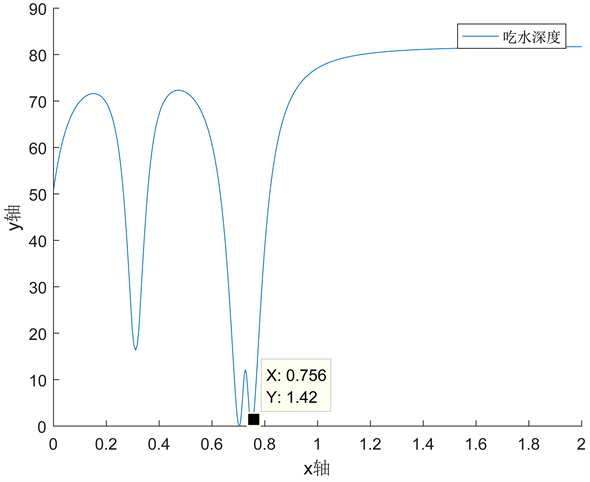Figure 10. The depth of the draft at 12 m/s (water velocity of 1.5 m/s in the same direction as the wind)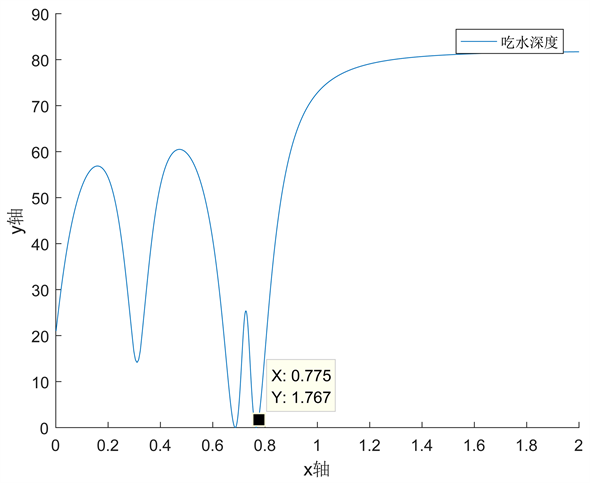Figure 11. The depth of the draft at 24 m/s (water velocity of 1.5 m/s in the same direction as the wind)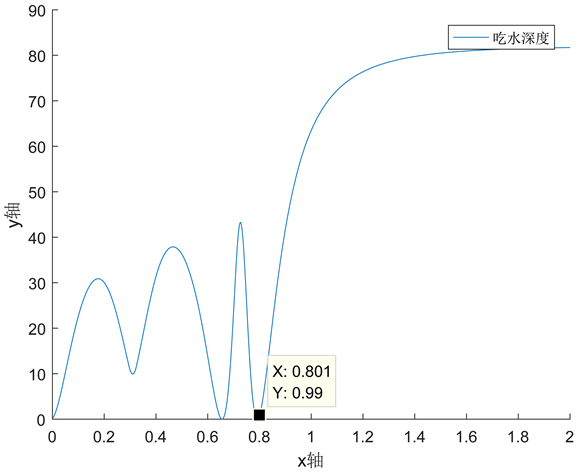Figure 12. The depth of the draft at 36 m/s (water velocity of 1.5 m/s in the same direction as the wind)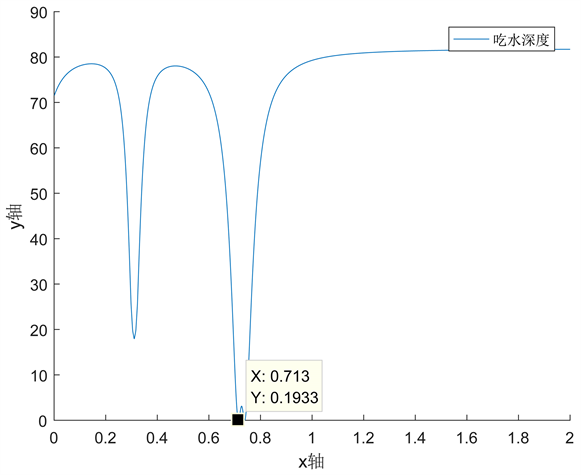Figure 13. The depth of the draft at 12 m/s (water velocity of 1.5 m/s in the different direction as the wind)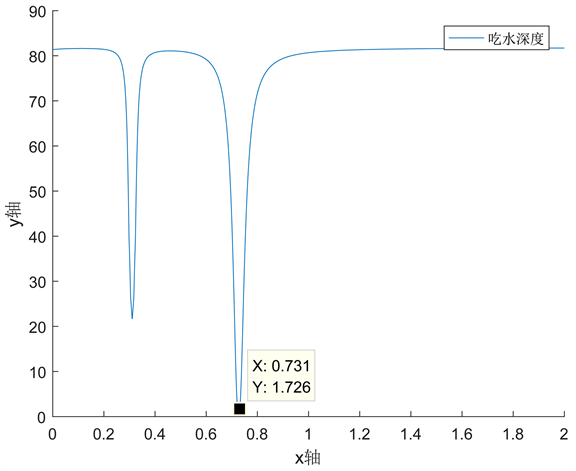Figure 14. The depth of the draft at 20 m/s (water velocity of 1.5 m/s in the different direction as the wind)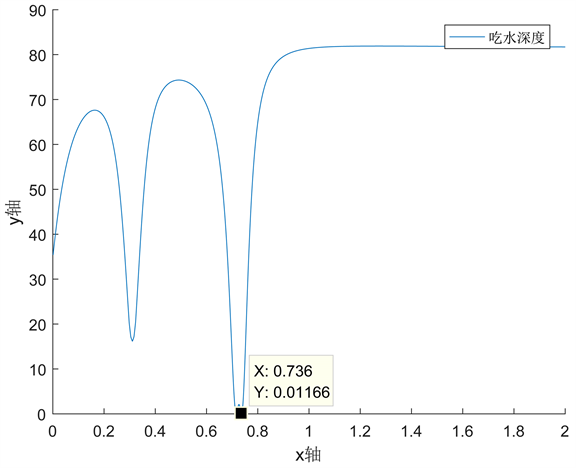Figure 15. The depth of the draft at 36 m/s (water velocity of 1.5 m/s in the different direction as the wind)Table 4. Draft depth and moving area of buoys at different wind speeds (water velocity of 1.5 m/s in the same direction as the wind)Table 5. The angle of inclination of the steel pipe and the angle between the tangent line and the vertical direction at the ends of the cable at different wind speeds (water velocity of 1.5 m/s in the same direction as the wind)Table 6. Draft depth and moving area of buoys at different wind speeds (water velocity of 1.5 m/s in the different direction as the wind)Table 7. The angle of inclination of the steel pipe and the angle between the tangent line and the vertical direction at the ends of the cable at different wind speeds (water velocity of 1.5 m/s in the different direction as the wind)

5. 模型的评价 

5.1. 模型的进步性

5.2. 模型的局限性

 罗晓健, 张爱霞, 李绂. 单点系泊系统设计思路及原则[J]. 中国海洋平台, 2013, 28(2): 19-23.

 王亚洲. 深海单点系泊海洋浮标锚泊系统研究[D]: [硕士学位论文]. 青岛: 中国海洋大学, 2013.

 杨长义, 陈玺文. 浅析系船浮筒锚链的静力计算问题[J]. 港工技术, 2012, 49(2): 18-20.

 肖越. 系泊系统时域非线性计算分析[D]: [博士学位论文]. 大连: 大连理工大学, 2006.

 夏运强, 王绿卿. 浮筒式防风单点系泊系统系泊线特性分析[A]. 全国结构工程学术会议, 2012.